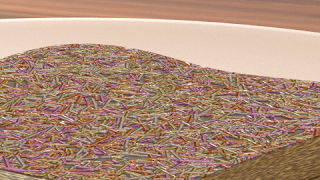### Sprinkles revisited

A while ago when I started this blog I created a simple shader for chocolate sprinkles and while I was reviewing comments I noticed that the code could do with some extra love.(Whether you think this is a tasty image probably depends on you being a five year old or not :-)
There are a couple of issues with the original code:
Unnecessary functions
When the code was written OSL was lacking an implementation of the function to calculate the distance to a line segment. This is no longer the case and the functionality is provided by the three argument version of the `distance()` function.
Unsophisticated use of cellnoise
In the original code we added all sorts of vectors to get more than one uncorrelated cell noise value but in fact all noise variants in OSL support 4 dimensions so we can use a vector plus an additional value to get different noise values for a given cell.
Use of a deprecated function
The `cellnoise()` function is deprecated and we should use `noise("cell", ...)` in stead.
Unnecessary include
`stdosl.h` is automatically included by Blender already so there is no need for us to include it again.
And as a commenter mentioned, the code could do with an extra output to identify individual sprinkles so we can color them.

## Code and node setup

The cleaned up code is pretty straight forward (and quite a bit shorter):
```shader sprinkles(
point Pos = P,
float Scale = 1,
int Np = 1,
int Seed = 42,
float Size = 1,
output float Fac = 0,
output float Random = 0
){
point p = Pos * Scale;
point f = floor(p);

int xx,yy,np;
vector one = 1;

for( xx=-1; xx<=1; xx++){
for( yy=-1; yy<=1; yy++){
point ff = f + vector(xx,yy,0);
float u=Seed;

for( np=0; np < Np; np++){
vector pd1 = 2*noise("cell",ff,u)-one;
vector pd2 = 2*noise("cell",ff,u+1)-one;

point p1 = ff + pd1;
point p2 = ff + pd2;

p2 = (p2 - p1)*Size+p1;

// reduce to 2D
p1=0;
p2=0;
p =0;

float r = distance(p1,p2,p);
if ( r < Radius ) {
Random = noise("cell",ff,u+2);
}
u+=3;
}
}
}
}```
The node setup used to make the image at the top looks like this:## Code availability

The shader is available on GitHub. If you would like to know more about programming OSL you might be interested in my book "Open Shading Language for Blender". More on the availability of this book and a sample can be found on this page.